# C# For loop with Examples

In c#, for loop is useful to execute a statement or a group of statements repeatedly until the defined condition returns true.

Generally, for loop is useful in c# applications to iterate and execute a certain block of statements repeatedly until the specified number of times.

## Syntax of C# For Loop

Following is the syntax of defining for loop in c# programming language.

for (initialization; condition; iterator(inc / dec))
{
// Statements to Execute
}

If you observe the above syntax, we defined a for loop with 3 parts: initialization, condition, iterator, and these are separated with a semicolon (;).

1. In the initialization part, the variable will be declared and initialized. The initialization part will be executed only once at the starting of the for loop.
2. After completion of the initialization part, the condition part will be evaluated. Here the condition is a boolean expression, and it will return either true or false.
3. In case if the condition is evaluated to true
• The statements inside of for loop will be executed.
• After that, the iterator part will be executed, and it will increase or decrease the initialized variable value based on our requirements.
• After changing the variable value, again, the condition will be evaluated, and execute the statements within the loop.
• This process will continue until the condition is evaluated as false.
4. If the condition is evaluated to false, then the for loop execution will be stopped, and control will come out of the loop.

For example, if we have a for loop to print the variable (i) value to 4 times, the process flow of for loop will be like as shown below.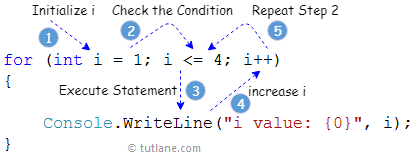As discussed, first we declared and assigned a value (1) to the variable i, then the condition (i <= 4) will be evaluated. Since the condition is true, then the statements within the loop will be executed.

After that, the iterator (i++) will be evaluated, and it will increase the value of a variable (i). Again the condition (i <= 4) checking will happen, and it will continue until the condition returns true.

Here the condition will return true till the variable i value becomes 4. After that, if i value becomes 5, then the condition (5 <= 4) will fail, and it returns false

## C# For Loop Flowchart Diagram

Following is the pictorial representation of for loop process flow diagram in the c# programming language.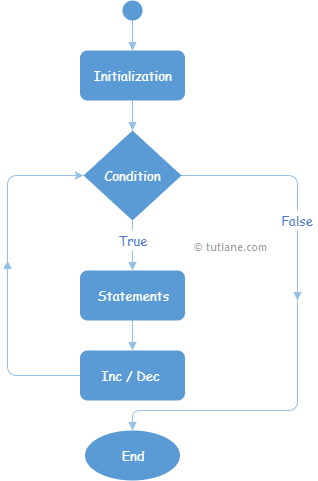Now we will see how to use for loop in c# programming language with examples.

## C# For Loop Example

Following is the example of using for loop in c# programming language to iterate or loop through a particular list of statements.

using System;

namespace Tutlane
{
class Program
{
static void Main(string[] args)
{
for (int i = 1; i <= 4; i++)
{
Console.WriteLine("i value: {0}", i);
}
Console.WriteLine("Press Enter Key to Exit..");
Console.ReadLine();
}
}
}

If you observe the above code, we defined a for loop to iterate over 4 times to print the value of variable i, and the following are the main parts of for loop.

• Here int i = 1 is the initialization part
• i <= 4 is the condition part
• i++ is the iterator part

When we execute the above c# program, we will get the result as shown below.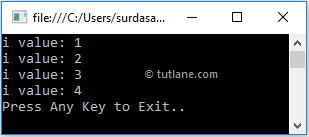If you observe the above result, for loop executed 4 times and printed the i variable value for 4 times.

## C# For Loop with Multiple Variables

In c# for loop, we can declare and initialize multiple variables and iterator expressions by separating with comma (,) operator.

Following is the example of using multiple variables and iterator expressions in c# for loop.

using System;

namespace Tutlane
{
class Program
{
static void Main(string[] args)
{
for (int i = 1, j = 0; i <= 4; i++, j++)
{
Console.WriteLine("i: {0}, j: {1}", i, j);
}
Console.WriteLine("Press Enter Key to Exit..");
Console.ReadLine();
}
}
}

If you observe the above example, we defined two variables (i, j) and two iterator expressions (i++, j++) by separating them with comma (,) operator.

When we execute the above c# program, we will get the result as shown below.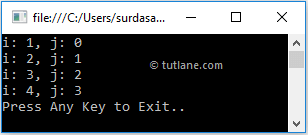This is how we can use multiple variables and multiple iterators in c# for loops based on our requirements.

## C# For Loop with Break Statement

In c#, by using the break keyword we can stop the execution of for loop statement based on our requirements.

Following is the example of stop the execution of for loop using the break statement.

using System;

namespace Tutlane
{
class Program
{
static void Main(string[] args)
{
for (int i = 1; i <= 4; i++)
{
if (i == 3)
break;
Console.WriteLine("i value: {0}", i);
}
Console.WriteLine("Press Enter Key to Exit..");
Console.ReadLine();
}
}
}

If you observe the above code, we used a break statement to exit for loop whenever the variable i value equals 3.

When we execute the above c# program, we will get the result as shown below.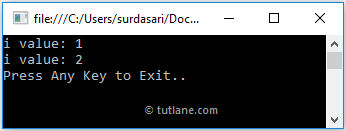If you observe the above result, the loop execution has stopped automatically whenever the variable i value equals 3.

This is how we can use the break statement in for loop to terminate the execution of for loop based on our requirements.

## C# For Loop without Initialization & Iterators

Generally, the initializer, condition, and iterator parameters are optional to create for loop in c# programming language.

Following is the example of creating a for loop in c# programming language without initializer and iterator.

using System;

namespace Tutlane
{
class Program
{
static void Main(string[] args)
{
int i = 1;
for ( ; i <= 4; )
{
i++;
Console.WriteLine("i value: {0}", i);
}
Console.WriteLine("Press Enter Key to Exit..");
Console.ReadLine();
}
}
}

If you observe the above example, we defined a for loop without any initializers and iterators.

When we execute the above c# program, we will get the result as shown below.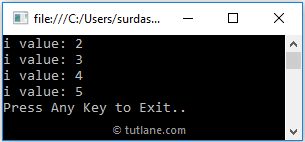This is how we can implement for loop in c# programming language without initializers and iterators based on our requirements.

## C# Infinite For Loop

If the condition parameter in for loop always returns true, the for loop will be infinite and runs forever. Even if we miss the condition parameter in for loop automatically, that loop will become an infinite loop.

The following are the different ways to make for loop an infinite loop in the c# programming language.

for (initializer; ; iterator) {
// Statements to Execute
}

or

for ( ; ; )
{
// Statements to Execute
}

Following is the example of making a for loop as an infinite in c# programming language.

using System;

namespace Tutlane
{
class Program
{
static void Main(string[] args)
{
for (int i = 1; i > 0; i++)
{
i++;
Console.WriteLine("i value: {0}", i);
}
Console.WriteLine("Press Enter Key to Exit..");
Console.ReadLine();
}
}
}

If you observe the above code, the condition (i > 0), whatever we defined in for loop, will always become true and return infinite results.

## C# Nested For Loop

In c#, we can create one for loop within another for loop based on our requirements. Following is the example of creating a nested for loop in the c# programming language.

using System;

namespace Tutlane
{
class Program
{
static void Main(string[] args)
{
for (int i = 1; i <= 4; i++)
{
for (int j = i; j < 3; j++)
{
Console.WriteLine("i value: {0}, j value: {1}", i, j);
}
}
Console.WriteLine("Press Enter Key to Exit..");
Console.ReadLine();
}
}
}

If you observe the above example, we created a for loop within another loop and printing the values based on our requirements.

When we execute the above c# program, we will get the result as shown below.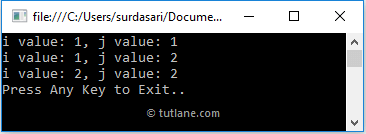This is how we can create nested for loops in our c# programming language based on our requirements.